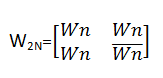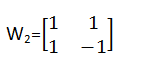# Code-Division Multiple Access (CDMA) in Computer Network

Computer Network | CDMA: In this tutorial, we will learn Code-Division Multiple Access (CDMA) and how to implement CDMA with Walsh table? By Radib Kar Last updated : May 05, 2023

## What is Code-Division Multiple Access (CDMA)?

The analogy behind code-division multiple access says there are four people and two out of them are talking with each other in some language what other two don't know and the same goes for those two also. For example, two of them talking in Punjabi and two in Bengali. Those two talking in Punjabi has no idea about Bengali and same for Bengali guys too. So, there is no interruption in their respective communication. The same analogy has been used for CDMA.

## Code-Division Multiple Access (CDMA) Example

We discuss the concept with the help of an example,

```Say there are four stations: A, B, C, D
Each station has assigned a code say C1, C2, C3, C4
Each of them sending their respective data say D1, D2, D3, D4
All of them throws their data multiplying with the code

Thus data on the channel is
C1D1 + C2D2 + C3D3 + C4D4
```

The receiver who wants to retrieve simply multiplying the data with its code and divide by the number of the station.

Now, this is possible because of the coding theory. Let's see the how codes are generated, encoding and decoding of data bit and rules of addition and multiplication

## CDMA Code Generation

Code generation is done by Walsh table

Walsh table is a kind of recursive table which is represented by

```Where W1= 
2N=No of stations
So, for 2 stationsCode for first station is [1 1] //vector
Code for second station is [1 -1] //vector

Additions and multiplications are vector scalar type
Say [1 0]*[-1 1]=(1*-1)+ (0*1)]=-1+0=-1
```

### Encoding and decoding of data

• If a station is sending bit 0, it's encoded as -1
• If a station is sending bit 1, it's encoded as 1
• If station is idle, it's encoded as 0

### Example with 2 stations

```Say, Station A sends 1, B sends 0
Code of A: [1 1]
Data of A: 1
Code of B: [1 -1]
Data of B: -1

Dara on channel
1*[1 1] + -1*[1 -1]
=[1 1] + [-1 1]
=[0 2] //scalar addition of vector

Now say A wants to retrieve its data
So multiply data on channel with A’s code
([0 2] * [1 1 ] )/2
=(0*1 + 2*1)=2/2=1

For B
([0 2] * [1 -1])/2=-2/2=-1
```

## Code-Division Multiple Access (CDMA) | C++ Implementation

```#include <bits/stdc++.h>

using namespace std;

class CDMA {
public: int ** wtable;
int ** copy;
int * channel_sequence;

void setUp(int data[], int num_stations) {
int n = num_stations;
wtable = new int * [n];

for (int i = 0; i < n; i++)
wtable[i] = new int[n];

copy = new int * [n];
for (int i = 0; i < n; i++)
copy[i] = new int[n];

buildWalshTable(num_stations, 0, num_stations - 1, 0, num_stations - 1, false);

showWalshTable(num_stations);

for (int i = 0; i < num_stations; i++) {
for (int j = 0; j < num_stations; j++) {
copy[i][j] = wtable[i][j];
wtable[i][j] *= data[i];
}
}

channel_sequence = new int[n];

for (int i = 0; i < num_stations; i++) {
for (int j = 0; j < num_stations; j++) {
channel_sequence[i] += wtable[j][i];
}
}
}

void listenTo(int sourceStation, int num_stations) {
int innerProduct = 0;
for (int i = 0; i < num_stations; i++) {
innerProduct += copy[sourceStation][i] * channel_sequence[i];
}

int k = innerProduct / num_stations;

if (k == 1)
cout << "The data received from station " << sourceStation + 1 << " is: " << k << endl;
else if (k == -1)
cout << "The data received from station " << sourceStation + 1 << " is: 0" << endl;
else
cout << "Station " << sourceStation + 1 << " is idle, it didn't send any data\n";
}

//building walsh table
int buildWalshTable(int len, int i1, int i2, int j1, int j2, bool isBar) {
if (len == 2) {
if (!isBar) {
wtable[i1][j1] = 1;
wtable[i1][j2] = 1;
wtable[i2][j1] = 1;
wtable[i2][j2] = -1;
} else {
wtable[i1][j1] = -1;
wtable[i1][j2] = -1;
wtable[i2][j1] = -1;
wtable[i2][j2] = +1;
}
return 0;
}

int midi = (i1 + i2) / 2;
int midj = (j1 + j2) / 2;

buildWalshTable(len / 2, i1, midi, j1, midj, isBar);
buildWalshTable(len / 2, i1, midi, midj + 1, j2, isBar);
buildWalshTable(len / 2, midi + 1, i2, j1, midj, isBar);
buildWalshTable(len / 2, midi + 1, i2, midj + 1, j2, !isBar);

return 0;
}

void showWalshTable(int num_stations) {
cout << "................Displaying walsh table..................\n";
//cout<<endl;
for (int i = 0; i < num_stations; i++) {
for (int j = 0; j < num_stations; j++) {
cout << wtable[i][j] << "  ";
}
cout << "\n";
}
cout << "----------------------------------------------------------\n";
}
};

int main() {
cout << "-------------------------------CDMA Implementation------------------------\n";

int num_stations;
cout << "Enter no of stations\n";

cin >> num_stations;
//data bits corresponding to each station
cout << "Press 1 if station is sending bit 1\n";
cout << "Press -1 if station is sending bit 0\n";
cout << "Press 0 if station is idle\n";
int * data = new int[num_stations];
for (int i = 0; i < num_stations; i++) {
cout << "enter for station " << i + 1 << endl;
cin >> data[i];
}

CDMA channel;

channel.setUp(data, num_stations);

// station you want to listen to
cout << "Enter station no you want to listen to\n";
int sourceStation;
cin >> sourceStation;

channel.listenTo(sourceStation - 1, num_stations);
return 0;
}
```

### Output 1

```-------------------------------CDMA Implementation------------------------
Enter no of stations
4
Press 1 if station is sending bit 1
Press -1 if station is sending bit 0
Press 0 if station is idle
enter for station 1
1
enter for station 2
0
enter for station 3
-1
enter for station 4
-1
................Displaying walsh table..................
1  1  1  1
1  -1  1  -1
1  1  -1  -1
1  -1  -1  1
----------------------------------------------------------
Enter station no you want to listen to
4
The data received from station 4 is: 0
```

### Output 2

```-------------------------------CDMA Implementation------------------------
Enter no of stations
8
Press 1 if station is sending bit 1
Press -1 if station is sending bit 0
Press 0 if station is idle
enter for station 1
1
enter for station 2
1
enter for station 3
-1
enter for station 4
-1
enter for station 5
0
enter for station 6
0
enter for station 7
-1
enter for station 8
1
................Displaying walsh table..................
1  1  1  1  1  1  1  1
1  -1  1  -1  1  -1  1  -1
1  1  -1  -1  1  1  -1  -1
1  -1  -1  1  1  -1  -1  1
1  1  1  1  -1  -1  -1  -1
1  -1  1  -1  -1  1  -1  1
1  1  -1  -1  -1  -1  1  1
1  -1  -1  1  -1  1  1  -1
----------------------------------------------------------
Enter station no you want to listen to
5
Station 5 is idle, it didn't send any data
```